# Lattice 2003 The XXI International Symposium on Lattice

• Slides: 18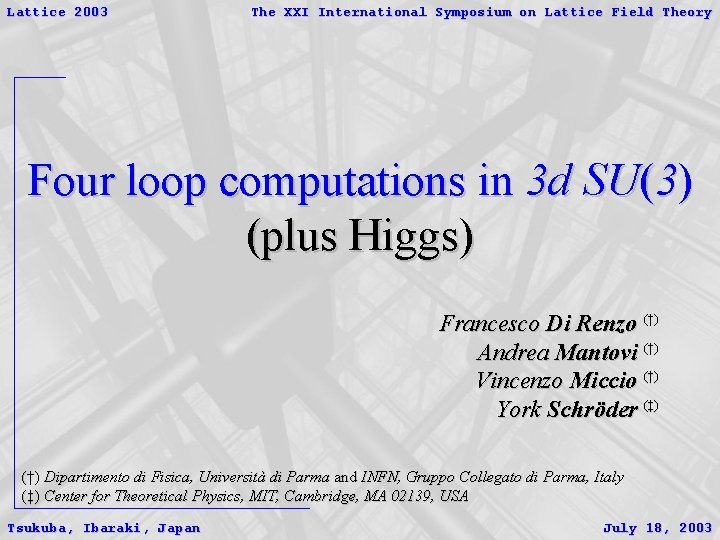Lattice 2003 The XXI International Symposium on Lattice Field Theory Four loop computations in 3 d SU(3) (plus Higgs) Francesco Di Renzo (†) Andrea Mantovi (†) Vincenzo Miccio (†) York Schröder (‡) (†) Dipartimento di Fisica, Università di Parma and INFN, Gruppo Collegato di Parma, Italy (‡) Center for Theoretical Physics, MIT, Cambridge, MA 02139, USA Tsukuba, Ibaraki, Japan July 18, 2003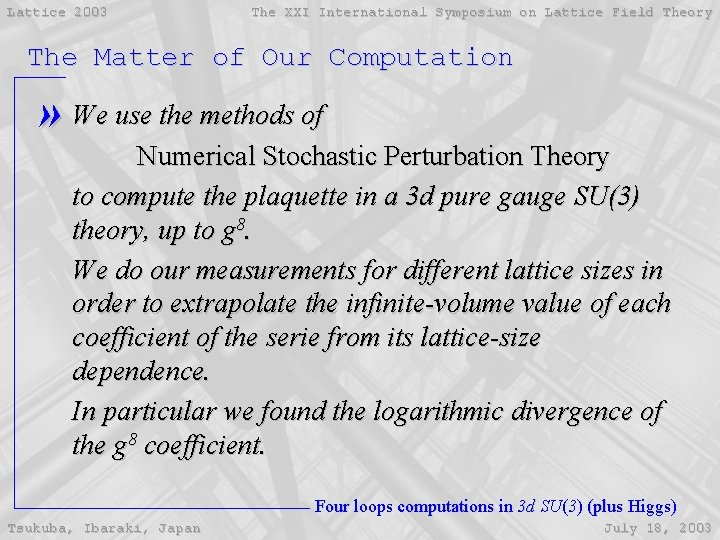Lattice 2003 The XXI International Symposium on Lattice Field Theory The Matter of Our Computation » We use the methods of Numerical Stochastic Perturbation Theory to compute the plaquette in a 3 d pure gauge SU(3) theory, up to g 8. We do our measurements for different lattice sizes in order to extrapolate the infinite-volume value of each coefficient of the serie from its lattice-size dependence. In particular we found the logarithmic divergence of the g 8 coefficient. Four loops computations in 3 d SU(3) (plus Higgs) Tsukuba, Ibaraki, Japan July 18, 2003Lattice 2003 The XXI International Symposium on Lattice Field Theory Outline » Sketch of Physical Framework » The Algorithm & The Code » Results in Pure Gauge Sector » Perspective in Complete Theory Four loops computations in 3 d SU(3) (plus Higgs) Tsukuba, Ibaraki, Japan July 18, 2003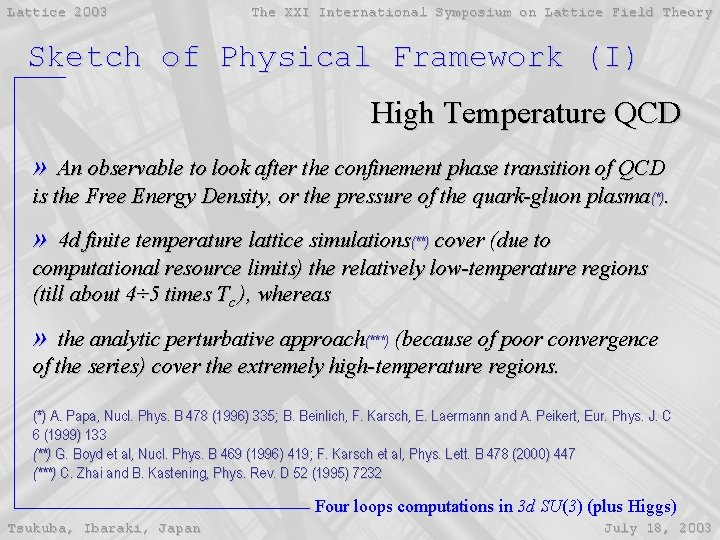Lattice 2003 The XXI International Symposium on Lattice Field Theory Sketch of Physical Framework (I) High Temperature QCD » An observable to look after the confinement phase transition of QCD is the Free Energy Density, or the pressure of the quark-gluon plasma(*). » 4 d finite temperature lattice simulations(**) cover (due to computational resource limits) the relatively low-temperature regions (till about 4÷ 5 times Tc ), whereas » the analytic perturbative approach(***) (because of poor convergence of the series) cover the extremely high-temperature regions. (*) A. Papa, Nucl. Phys. B 478 (1996) 335; B. Beinlich, F. Karsch, E. Laermann and A. Peikert, Eur. Phys. J. C 6 (1999) 133 (**) G. Boyd et al, Nucl. Phys. B 469 (1996) 419; F. Karsch et al, Phys. Lett. B 478 (2000) 447 (***) C. Zhai and B. Kastening, Phys. Rev. D 52 (1995) 7232 Four loops computations in 3 d SU(3) (plus Higgs) Tsukuba, Ibaraki, Japan July 18, 2003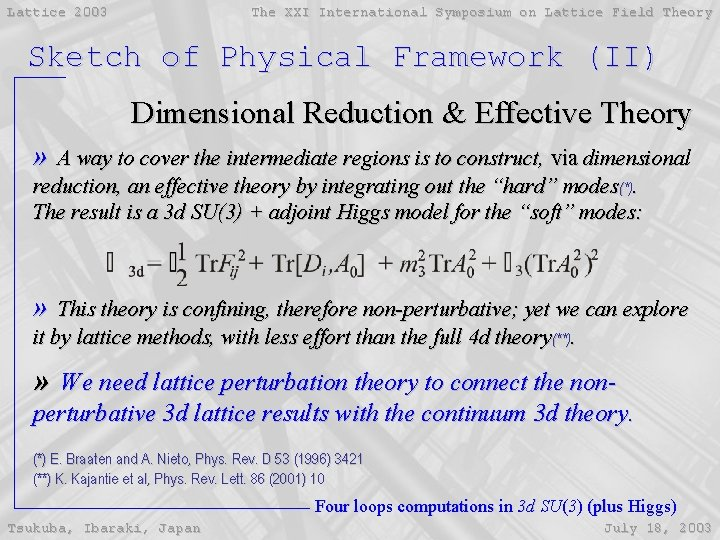Lattice 2003 The XXI International Symposium on Lattice Field Theory Sketch of Physical Framework (II) Dimensional Reduction & Effective Theory » A way to cover the intermediate regions is to construct, via dimensional reduction, an effective theory by integrating out the “hard” modes(*). The result is a 3 d SU(3) + adjoint Higgs model for the “soft” modes: » This theory is confining, therefore non-perturbative; yet we can explore it by lattice methods, with less effort than the full 4 d theory(**). » We need lattice perturbation theory to connect the nonperturbative 3 d lattice results with the continuum 3 d theory. (*) E. Braaten and A. Nieto, Phys. Rev. D 53 (1996) 3421 (**) K. Kajantie et al, Phys. Rev. Lett. 86 (2001) 10 Four loops computations in 3 d SU(3) (plus Higgs) Tsukuba, Ibaraki, Japan July 18, 2003Lattice 2003 The XXI International Symposium on Lattice Field Theory The Algorithm (I) Stochastic Quantization(*) » A stochastic dynamical system on the field configuration space dictated by the general Langevin equation such that averages on the noise h converge to averages on the Gibbs measure: (*) G. Parisi and Wu Yongshi, Sci. Sinica 24 (1981) 35 Four loops computations in 3 d SU(3) (plus Higgs) Tsukuba, Ibaraki, Japan July 18, 2003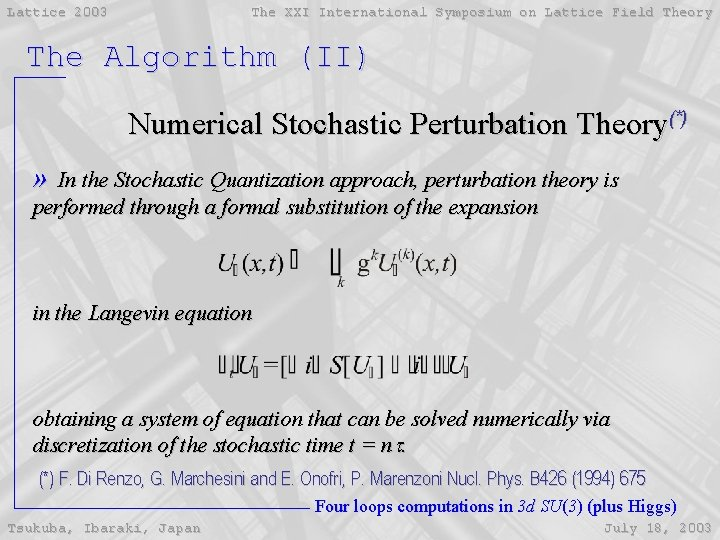Lattice 2003 The XXI International Symposium on Lattice Field Theory The Algorithm (II) Numerical Stochastic Perturbation Theory(*) » In the Stochastic Quantization approach, perturbation theory is performed through a formal substitution of the expansion in the Langevin equation obtaining a system of equation that can be solved numerically via discretization of the stochastic time t = nt. (*) F. Di Renzo, G. Marchesini and E. Onofri, P. Marenzoni Nucl. Phys. B 426 (1994) 675 Four loops computations in 3 d SU(3) (plus Higgs) Tsukuba, Ibaraki, Japan July 18, 2003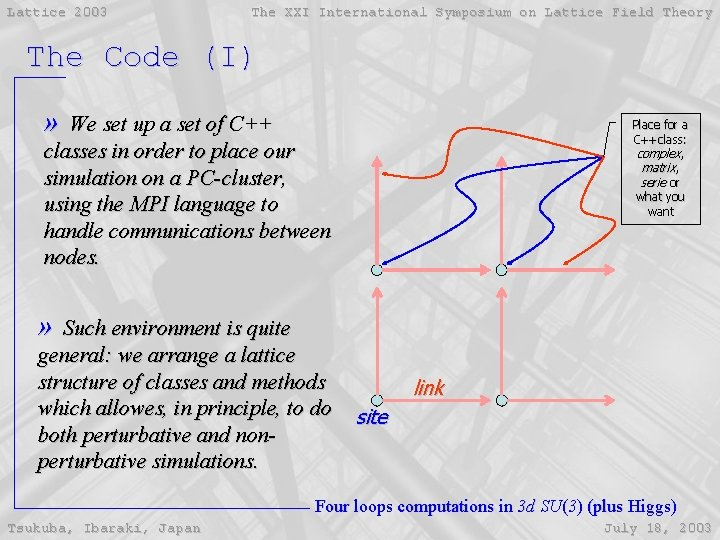Lattice 2003 The XXI International Symposium on Lattice Field Theory The Code (I) » We set up a set of C++ classes in order to place our simulation on a PC-cluster, using the MPI language to handle communications between nodes. Place for a C++class: complex, matrix, serie or what you want » Such environment is quite general: we arrange a lattice structure of classes and methods which allowes, in principle, to do both perturbative and nonperturbative simulations. link site Four loops computations in 3 d SU(3) (plus Higgs) Tsukuba, Ibaraki, Japan July 18, 2003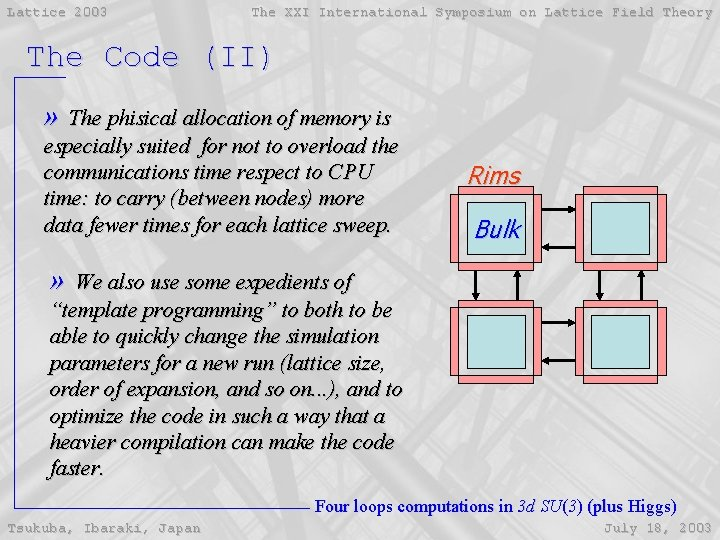Lattice 2003 The XXI International Symposium on Lattice Field Theory The Code (II) » The phisical allocation of memory is especially suited for not to overload the communications time respect to CPU time: to carry (between nodes) more data fewer times for each lattice sweep. Rims Bulk » We also use some expedients of “template programming” to both to be able to quickly change the simulation parameters for a new run (lattice size, order of expansion, and so on. . . ), and to optimize the code in such a way that a heavier compilation can make the code faster. Four loops computations in 3 d SU(3) (plus Higgs) Tsukuba, Ibaraki, Japan July 18, 2003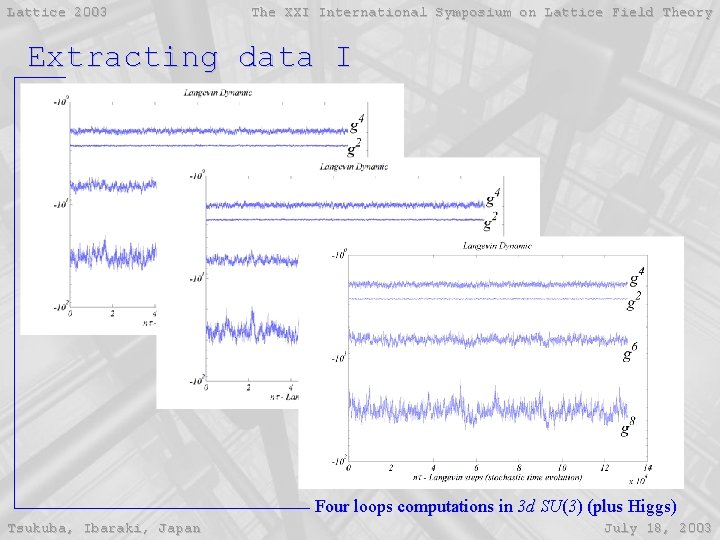Lattice 2003 The XXI International Symposium on Lattice Field Theory Extracting data I Four loops computations in 3 d SU(3) (plus Higgs) Tsukuba, Ibaraki, Japan July 18, 2003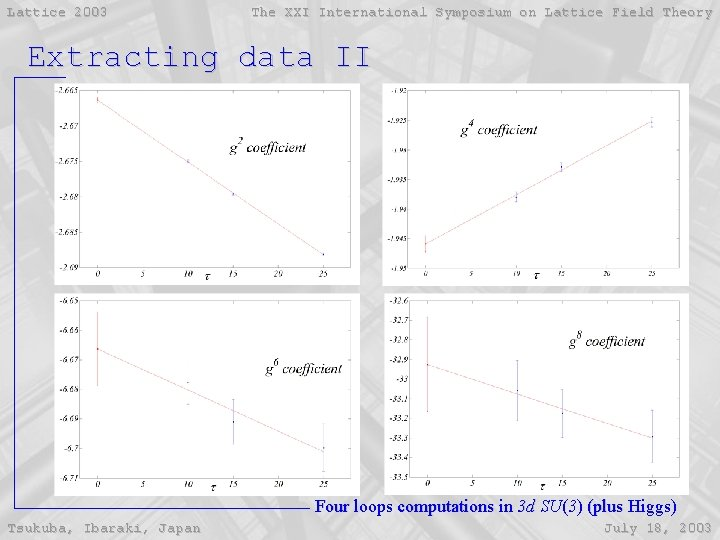Lattice 2003 The XXI International Symposium on Lattice Field Theory Extracting data II Four loops computations in 3 d SU(3) (plus Higgs) Tsukuba, Ibaraki, Japan July 18, 2003Lattice 2003 The XXI International Symposium on Lattice Field Theory Results Four loops computations in 3 d SU(3) (plus Higgs) Tsukuba, Ibaraki, Japan July 18, 2003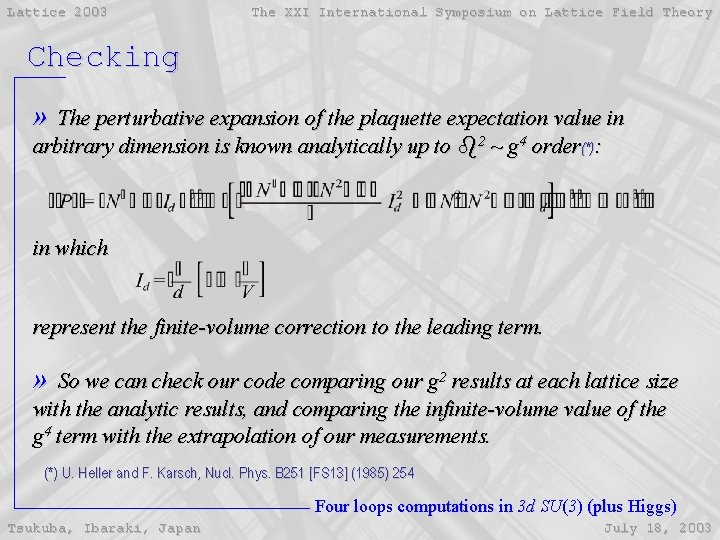Lattice 2003 The XXI International Symposium on Lattice Field Theory Checking » The perturbative expansion of the plaquette expectation value in arbitrary dimension is known analytically up to b 2 ~ g 4 order(*): in which represent the finite-volume correction to the leading term. » So we can check our code comparing our g 2 results at each lattice size with the analytic results, and comparing the infinite-volume value of the g 4 term with the extrapolation of our measurements. (*) U. Heller and F. Karsch, Nucl. Phys. B 251 [FS 13] (1985) 254 Four loops computations in 3 d SU(3) (plus Higgs) Tsukuba, Ibaraki, Japan July 18, 2003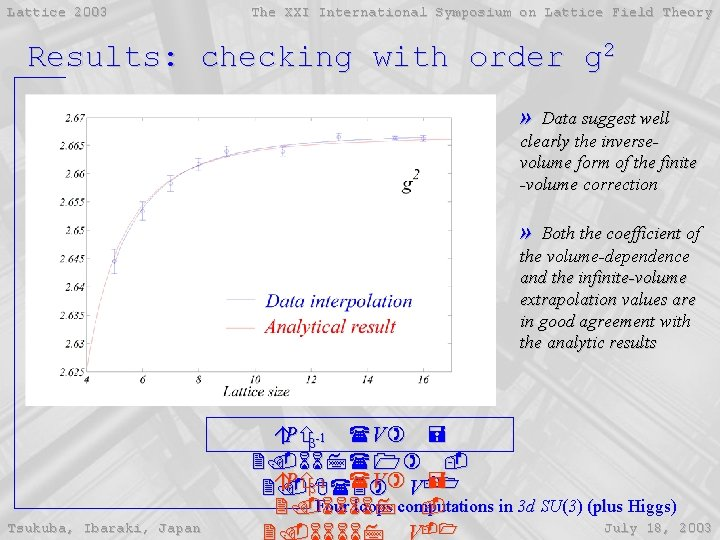Lattice 2003 The XXI International Symposium on Lattice Field Theory Results: checking with order g 2 » Data suggest well clearly the inversevolume form of the finite -volume correction » Both the coefficient of the volume-dependence and the infinite-volume extrapolation values are in good agreement with the analytic results áPñb-1 (V) = 2. 667(1) áPñb-1 (V) V-1 = 2. 8(3) Four loops computations in 3 d SU(3) (plus Higgs) 2. 66667 Tsukuba, Ibaraki, Japan -1 July 18, 2003Lattice 2003 The XXI International Symposium on Lattice Field Theory Results: checking with order g 4 » Different power laws for size-dependence are tried in the interpolation (as “effective” finitesize correction), leading to quite the same infinite -volume extrapolation » The value found is again in good agreement with the analytic result áPñb-2(V=¥) = 1. 95(1) áPñb-2(V=¥) = 1. 94862 Four loops computations in 3 d SU(3) (plus Higgs) Tsukuba, Ibaraki, Japan July 18, 2003Lattice 2003 The XXI International Symposium on Lattice Field Theory Results: order g 6 » Again we fit different power-law for the finitesize dependence in order to extract the asintotic value » In (*), they estimate this coefficient rescaling the analogous 4 d value which is known(**). The number they use áPñb-3 » 7. 02 is in quite well agreement with the value we found áPñb-3(V=¥) = 6. 7(2) (*) F. Karsch, M. Lütgemeier, A. Patkòs and J. Rank, Phys. Lett. B 390, 275 (1997) (**) B. Allés, M. Campostrini, A. Feo and H. Panagopoulos, Phys. Lett. B 324 (1994) 433 Four loops computations in 3 d SU(3) (plus Higgs) Tsukuba, Ibaraki, Japan July 18, 2003Lattice 2003 The XXI International Symposium on Lattice Field Theory Results: order g 8 » For this coefficient we expect a logarithmic divergence in the lattice volume(*). Indeed data fit better if we add a log-term in the interpolation-law » The coefficient of the ln. V divergence must be the same as the continuum log-divergence in the cut-off of the dimensional-reduced theory » So we can do another indirect check comparing the expected value(**) (0. 9765) with our result » If we use this analytical value in our fit, the estimate for the constat áPñb-4 (V) = 0. 9(4) coefficient improves, leading to the - result value of 25(2) ln(L 3) + 23(5) + k ck (L S (*) F. Karsch, M. Lütgemeier, A. k. Patkòs ) and J. Rank, Phys. Lett. B 390, 275 (1997) (**) K. Kajantie, M. Laine, K. Rummukainen, Y. Schroder, Phys. Rev. D 67, 105008 (2003) Four loops computations in 3 d SU(3) (plus Higgs) Tsukuba, Ibaraki, Japan July 18, 2003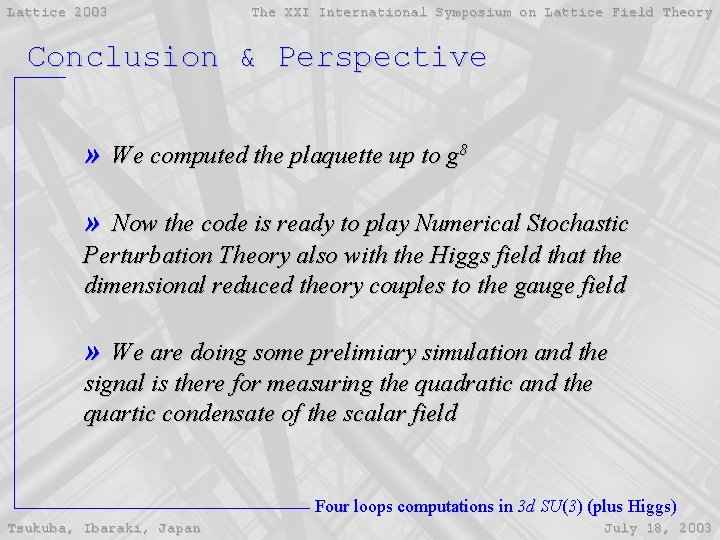Lattice 2003 The XXI International Symposium on Lattice Field Theory Conclusion & Perspective » We computed the plaquette up to g 8 » Now the code is ready to play Numerical Stochastic Perturbation Theory also with the Higgs field that the dimensional reduced theory couples to the gauge field » We are doing some prelimiary simulation and the signal is there for measuring the quadratic and the quartic condensate of the scalar field Four loops computations in 3 d SU(3) (plus Higgs) Tsukuba, Ibaraki, Japan July 18, 2003